# The PANEL Procedure

### Lagrange Multiplier (LM) Tests for Cross-Sectional and Time Effects

Subsections:

For random one-way and two-way error component models, the Lagrange multiplier test for the existence of cross-sectional or time effects or both is based on the residuals from the restricted model (that is, the pooled model). For more information about the Breusch-Pagan LM test, see the section Specification Tests.

#### Honda (1985) and Honda (1991) UMP Test and Moulton and Randolph (1989) SLM Test

The Breusch-Pagan LM test is two-sided when the variance components are nonnegative. For a one-sided alternative hypothesis, Honda (1985) suggests a uniformly most powerful (UMP) LM test for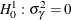(no cross-sectional effects) that is based on the pooled estimator. The alternative is the one-sided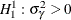. Let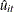be the residual from the simple pooled OLS regression and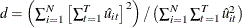. Then the test statistic is defined as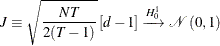The square of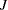is equivalent to the Breusch and Pagan (1980) LM test statistic. Moulton and Randolph (1989) suggest an alternative standardized Lagrange multiplier (SLM) test to improve the asymptotic approximation for Honda’s one-sided LM statistic. The SLM test’s asymptotic critical values are usually closer to the exact critical values than are those of the LM test. The SLM test statistic standardizes Honda’s statistic by its mean and standard deviation. The SLM test statistic is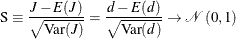Let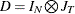, where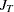is the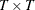square matrix of 1s. The mean and variance can be calculated by the formulas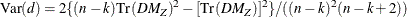where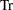denotes the trace of a particular matrix,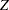represents the regressors in the pooled model,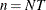is the number of observations, k is the number of regressors, and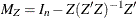. To calculate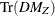, let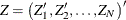. Then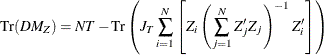To test for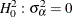(no time effects), define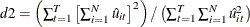. Then the test statistic is modified as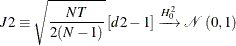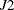can be standardized by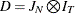, and other parameters are unchanged. Therefore,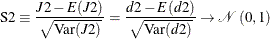To test for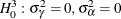(no cross-sectional and time effects), the test statistic is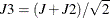and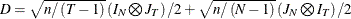. To standardize, define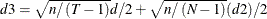,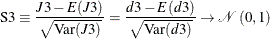#### King and Wu (1997) LMMP Test and the SLM Test

King and Wu (1997) derive the locally mean most powerful (LMMP) one-sided test for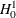and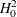, which coincides with the Honda (1985) UMP test. Baltagi, Chang, and Li (1992) extend the King and Wu (1997) test for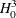as follows: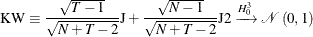For the standardization, use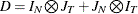. Define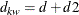; then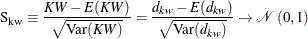#### Gourieroux, Holly, and Monfort (1982) LM Test

If one or both variance components (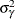and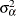) are small and close to 0, the test statisticsandcan be negative. Baltagi, Chang, and Li (1992) follow Gourieroux, Holly, and Monfort (1982) and propose a one-sided LM test for, which is immune to the possible negative values ofand. The test statistic is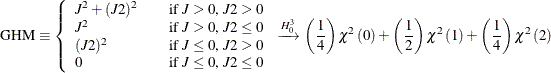where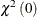is the unit mass at the origin.